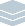Wolfram Archive
Wolfram Programming Lab is a legacy product.
All the same functionality and features, including access to Programming Lab Explorations, are available with Wolfram|One.
Start programming now. »
Try it now »
(no sign-in required)# Draw Circles of Points

Make simple figures based on points evenly spaced around a circle.

Run the code to draw a circle of 12 points. Try other numbers of points:

CirclePoints gives the coordinates of points evenly spaced around a circle. This gives 6 evenly spaced points:

CirclePoints

Draw the points with Graphics:

Graphics[Point[CirclePoints]]

Draw more points:

Graphics[Point[CirclePoints]]

Graphics[Point[CirclePoints]]

Draw a 12-sided polygon. Try other numbers of points:

Replace Point with Polygon to draw a 12-sided polygon:

Graphics[Polygon[CirclePoints]]

Graphics[Polygon[CirclePoints]]

Make it interactive. Drag the slider to change the shape:

Here is the code to draw a 12-sided polygon:

Graphics[Polygon[CirclePoints]]

Give yourself a slider to control the number of points using Manipulate. Wrap the expression with Manipulate[...], replace 12 with the variable n, and let n vary from 3 to 12 in steps of 1:

Manipulate[Graphics[Polygon[CirclePoints[n]]], {n, 3, 12, 1}]

Manipulate[Graphics[Polygon[CirclePoints[n]]], {n, 3, 12, 1}]